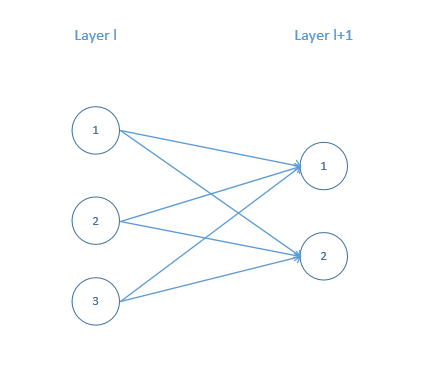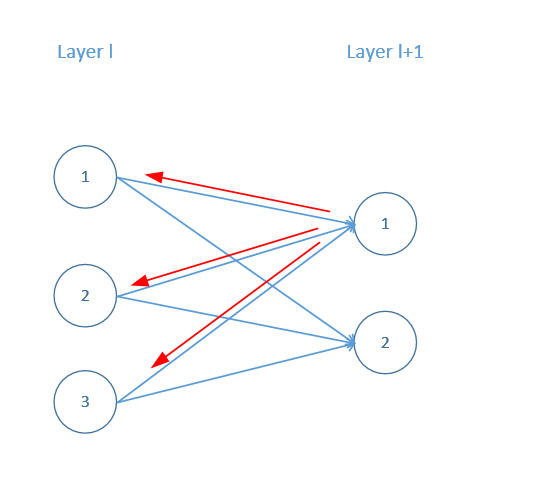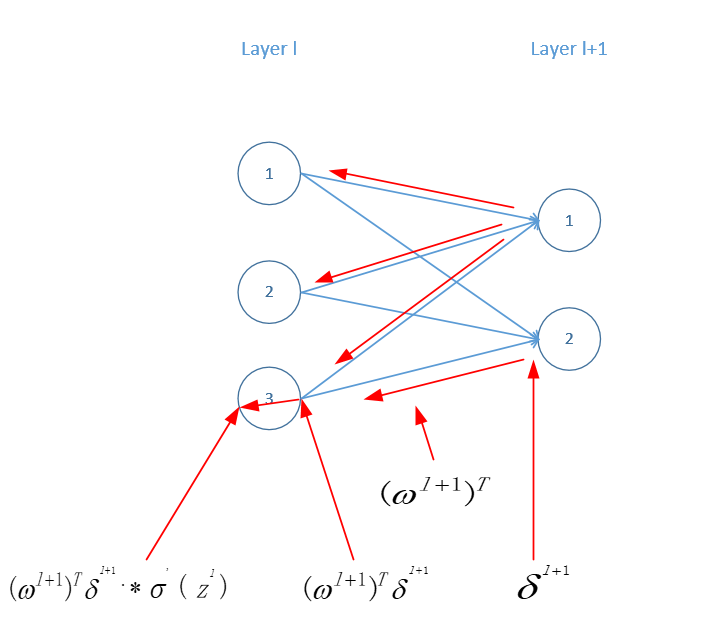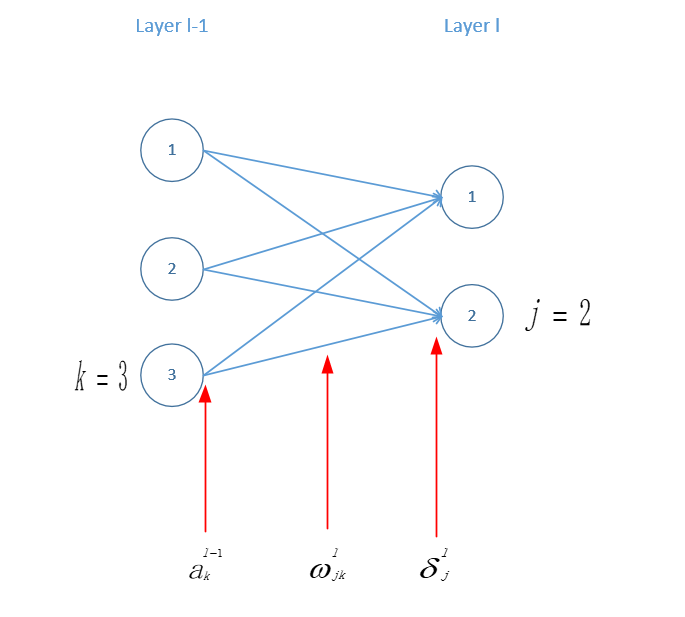• 两个假设
• 一个中间变量
• 四个公式

# 代价函数

C=12nx||y(x)aL(x)||2()

# 两个假设

1. 总体的代价函数可以写成全部单个样本的代价总和的平均值。
也就是C=1nxCx$C = \frac{1}{n}\sum_{x}C_{x}$，其中Cx=12||yaL||2$C_{x} = \frac{1}{2}{||y - a^L||}^2$

2. 代价函数可以认为是神经网络输出的函数。
比如对于单独的一个样本来说，代价函数可以写成C=12||yaL||=12j(yjaLj)2$C = \frac{1}{2}||y - a^L|| = \frac{1}{2}\sum_j{(y_j - {a^L}_j)}^2$

δlj=Czlj()

# 四个公式

### BP1:输出层误差公式

δLj=Caljσ(zLj)(BP-1)

δLj=aCσ(zLj)(BP-1a)

δLj=(aLy)σ(zLj)(BP-1b)

• 我们之前定义了中间变量 δlj=Czlj${{\delta}^l}_j = \frac{\partial C}{\partial {z^l}_j}$
因此对于最后一层（输出层）则有 δLj=CzLj${{\delta}^L}_j = \frac{\partial C}{\partial {z^L}_j}$
• C$C$aL$a^L$的函数(第二个假设C=12||yaL||=12j(yjaLj)2$C = \frac{1}{2}||y - a^L|| = \frac{1}{2}\sum_j{(y_j - {a^L}_j)}^2$)，而a$a$z$z$的函数(ali=σ(zli)${a^l}_i = \sigma({z^l}_i)$)，根据链式求导法则有δLj=kCaLkaLkzLj${{\delta}^L}_j = \sum_k \frac{\partial C}{\partial {a^L}_k} \frac{\partial {a^L}_k}{\partial {z^L}_j}$;
• 为什么会有一个k$\sum_k$呢？这是由于第二个假设C$C$可以看成aL$a^L$的函数C=12||yaL||=12j(yjaLj)2$C = \frac{1}{2}||y - a^L|| = \frac{1}{2}\sum_j{(y_j - {a^L}_j)}^2$，也就是C$C$是由L层全部的输出构成的，因此在求导的时候需要对每一个L层的激励元进行求导，假设L层有k个，再加上链式求导法则于是CzLj$\frac{\partial C}{\partial {z^L}_j}$就写成kCaLkaLkzLj$\sum_k \frac{\partial C}{\partial {a^L}_k} \frac{\partial {a^L}_k}{\partial {z^L}_j}$;
• 要注意aLk${a^L}_k$zLj${z^L}_j$的关系，一个是L层某个神经元的输出部分，一个是L层某个神经元的输入部分，只当这两个部分作用于同一神经元的时候才有意义，也就是k=j的时候aLk=σ(zLj)${a^L}_k = \sigma({z^L}_j)$这个式子才成立，或者说对于L层的第k个神经元来说，连到其他神经元的输入对它的输出没有意义啊，还可以这么理解对于z = x + 1 这样一个函数来说，你非要对y（一个不存在的自变量）求导（这个在复合函数求导中特别常见，经过第一次求偏导之后，第二次求偏导的自变量就没了因此得0），所以对于kj$k \not= j$aLkzLj$\frac{\partial {a^L}_k}{\partial {z^L}_j}$都等于0，所以最后式子化为δLj=CaLjaLjzLj${{\delta}^L}_j = \frac{\partial C}{\partial {a^L}_j} \frac{\partial {a^L}_j}{\partial {z^L}_j}$;
• 又因为aLj=σ(zLj)${a^L}_j = \sigma({z^L}_j)$，所以式子就变成δLj=CaLjσ(zLj)${{\delta}^L}_j = \frac{\partial C}{\partial {a^L}_j} \sigma'({z^L}_j)$ 这就是BP-1，BP-1是分量（elementwise）形式的表达方式。
再进一步由于C=12j(yjaLj)2$C = \frac{1}{2}\sum_j{(y_j - {a^L}_j)}^2$，所以CaLj=j(yjaLj)=j(aLjyj)$\frac{\partial C}{\partial {a^L}_j} = - \sum_j(y_j -{a^L}_j) = \sum_j({a^L}_j - y_j)$，所以BP-1又可以写成δLj=j(aLjyj)σ(zLj)${{\delta}^L}_j = \sum_j({a^L}_j - y_j){\sigma}^{'}({z^L}_j)$,这个就是BP-1b的分量形式。
• BP-1a是BP-1的向量形式，aC$\nabla_aC$表示C$C$a$a$上的梯度，$\nabla$是梯度符号，梯度是一个向量，其中的每个分量就是CaLj$\frac{\partial C}{\partial {a^L}_j}$

Calj$\frac{\partial C}{\partial {a^l}_j}$表示的是代价在第L层第j个神经元输出上的变化速度，如果C不太依赖这个神经元，那么Calj$\frac{\partial C}{\partial {a^l}_j}$的值理论上接近0，也就是整个式子为0，也就是说这个神经元基本上没有误差，是非常理想的情况。而σ(zLj)${\sigma}^{'}({z^L}_j)$提现了这个激活函数在zLj${z^L}_j$这一点上的变化速度。

### BP2：相邻两层之间的误差关系

δl=((wl+1)Tδl+1))σ(zl)(BP2)

• 我们还是中间变量开始δlj=Czlj${{\delta}^l}_j = \frac{\partial C}{\partial {z^l}_j}$
• 这一步利用了一些数学技巧：对于第l+1层来说有δl+1k=Czlj${{\delta}^{l+1}}_k = \frac{\partial C}{\partial {z^l}_j}$，我们可以推导出，第l层的第j个神经元上的输入zlj${z^l}_j$与第l+1层的的第k个神经元的输入zl+1k${z^{l+1}}_k$之间存在着这样的关系，zl+1k=jwl+1kjσ(zlj)+bl+1k${z^{l+1}}_k = \sum_j {w^{l+1}}_{kj}\sigma({z^l}_j) + {b^{l+1}}_k$，于是zl+1k${z^{l+1}}_k$可以看成zlj${z^l}_j$的函数，根据链式求导法则，我们重新改写一下δlj=Czlj${{\delta}^l}_j = \frac{\partial C}{\partial {z^l}_j}$这个公式为δlj=Czlj=kCzl+1kzl+1kzlj${{\delta}^l}_j = \frac{\partial C}{\partial {z^l}_j} = \sum_k \frac{\partial C}{\partial {z^{l+1}}_k}\frac{\partial {z^{l+1}}_k}{\partial {z^l}_j}$，而δl+1k=Czlj${{\delta}^{l+1}}_k = \frac{\partial C}{\partial {z^l}_j}$，于是kCzl+1kzl+1kzlj=kzl+1kzljδl+1k$\sum_k \frac{\partial C}{\partial {z^{l+1}}_k}\frac{\partial {z^{l+1}}_k}{\partial {z^l}_j} = \sum_k \frac{\partial {z^{l+1}}_k}{\partial {z^l}_j}{\delta^{l+1}_k}$
• zl+1kzlj=wl+1kjσ(zlj)$\frac{\partial {z^{l+1}}_k}{\partial {z^l}_j} = {w^{l+1}}_{kj}{\sigma}'({z^l}_j)$
为什么zl+1k${z^{l+1}}_k$明明是求和公式怎么一求偏导就没有累加符号了？符号太多看着有点眼晕，那么我们用一个简化问题的大招——代几个小数进去把问题具体化再观察其规律。
比如这样的一个结构j在l层，其取值范围为{1,2,3}，k在l+1层，取值范围为{1,2}，那么zl+1k=jwl+1kjσ(zlj)+bl+1k=wl+1k1σ(zl1)+wl+1k2σ(zl2)+wl+1k3σ(zl3)+bl+1k${z^{l+1}}_k = \sum_j {w^{l+1}}_{kj}\sigma({z^l}_j) + {b^{l+1}}_k = {w^{l+1}}_{k1}\sigma({z^l}_1) + {w^{l+1}}_{k2}\sigma({z^l}_2) + {w^{l+1}}_{k3}\sigma({z^l}_3) + {b^{l+1}}_k$，对于zl+1kzlj$\frac{\partial {z^{l+1}}_k}{\partial {z^l}_j}$我们令j=1$j=1$那么zl+1kzl1$\frac{\partial {z^{l+1}}_k}{\partial {z^l}_1}$相当于用上面展开的式子对zl1${z^l}_1$求偏导，那么对于不含有zl1${z^l}_1$的项自然为零了，结果就是wl+1k1σ(zl1)${w^{l+1}}_{k1}\sigma({z^l}_1)$
所以zl+1kzlj=wl+1kjσ(zlj)$\frac{\partial {z^{l+1}}_k}{\partial {z^l}_j} = {w^{l+1}}_{kj}{\sigma}'({z^l}_j)$
• 再回到式子kzl+1kzljδl+1k$\sum_k \frac{\partial {z^{l+1}}_k}{\partial {z^l}_j}{\delta^{l+1}_k}$中把上一步的结果代入，得到δlj=kwl+1kjσ(zlj)${{\delta}^l}_j = \sum_k {w^{l+1}}_{kj}{\sigma}'({z^l}_j)$，这个就是公式BP-2的分量形式。### BP3: 代价函数对任意一个神经元的偏置的偏导：

Cblj=δlj(BP3)

• 之前我们在推导相邻两层误差关系的时候利用了这个公式zl+1k=jwl+1kjσ(zlj)+bl+1k${z^{l+1}}_k = \sum_j {w^{l+1}}_{kj}\sigma({z^l}_j) + {b^{l+1}}_k$，所以有zlj=kwljkσ(zl1k)+blj${z^{l}}_j = \sum_k {w^{l}}_{jk}\sigma({z^{l-1}}_k) + {b^{l}}_j$，或者zlj=kwljkal1k+blj${z^{l}}_j = \sum_k {w^{l}}_{jk}{a^{l-1}}_k + {b^{l}}_j$（这里把l+1换成l，把j，k调换一下看着方便）。由这个公式我们可以知道zlj${z^l}_j$blj${b^l}_j$的函数。
• 我们依然利用链式求导法则改写Cblj$\frac{\partial C}{\partial {b^l}_j}$，则有Cblj=Czljzljblj$\frac{\partial C}{\partial {b^l}_j} = \frac{\partial C}{\partial {z^l}_j} \frac{\partial {z^l}_j }{\partial {b^l}_j}$
• 注意到Czlj$\frac{\partial C}{\partial {z^l}_j}$其实就是δlj${\delta^l}_j$ ,而zlj${z^l}_j$blj${b^l}_j$为1，也就是$\sum$那一大堆被当成常数求导为0。所以Cblj=δlj$\frac{\partial C}{\partial {b^l}_j} = {{\delta}^l}_j$

### BP4:代价函数对任意一个权重的偏导

Cwljk=al1kδlj(BP4)

• 我们依然使用 zlj=kwljkal1k+blj$z^{l}_j = \sum_k{w^{l}}_{jk}{a^{l-1}}_k + {b^{l}}_j$ 这个公式，这个公式我们可以知道zlj${z^l}_j$wljk${w^l}_{jk}$的函数
• 于是Cwljk=al1kδlj$\frac{\partial C}{\partial {w^l}_{jk}} = {a^{l-1}}_k{\delta^l}_j$。注意这里依然涉及到累和求导的问题，最后只会留下一个。Cwljk=al1kδlj$\frac{\partial C}{\partial {w^l}_{jk}} = {a^{l-1}}_k{\delta^l}_j$这个公式的下标太多以至于让人觉得很乱，于是我们还是用上面一个简单的结构具体化一下，最后我们再推出一般的情况。

### 小结一下反向传播的四个方程式

δLj=aCσ(zLj)(BP-1a)

δl=((wl+1)Tδl+1))σ(zl)(BP2)

Cblj=δlj(BP3)

Cwljk=al1kδlj(BP4)

# 反向传播算法

1. 输入x : 求输入层神经元的输出a1$a^1$ （这个一般来说就是样本输入）
2. 向前传播： 从第二层开始到最后一层，依次的计算各层的zl$z^l$al$a^l$
for l$l$ in [2,L]
compute zl$z^l$ (zl=wlal1+bl$z^l = w^la^{l-1}+b^l$)
compute al$a^l$ (al=σ(zl)$a^l = \sigma(z^l)$)
3. 计算输出层误差： 利用公式δLj=aCσ(zLj)${{\delta}^L}_j = \nabla_aC\bigodot{\sigma}^{'}({z^L}_j)$计算出输出层的误差
4. 反向传播误差：从L-1层开始一直到第二层，利用公式δl=((wl+1)Tδl+1))σ(zl)$\delta^l = ({(w^{l+1})}^T\delta^{l+1}))\bigodot{\sigma}^{'}({z^l})$计算每一层的误差
5. 输出：利用公式Cblj=δlj$\frac{\partial C}{\partial {b^l}_j} = {{\delta}^l}_j$Cwljk=al1kδlj$\frac{\partial C}{\partial {w^l}_{jk}} = {a^{l-1}}_k{\delta^l}_j$ 计算代价函数对所有未知参数的偏导数

# 参考文献：05-151458

#### 神经网络中的反向传播----Back Propagation

04-131385

#### 反向传播算法的理解

05-184483

#### 反向传播

05-273630

#### 【机器学习】彻底理解神经网络中的反向传播算法（BP）

11-053万+

#### 反向传播算法的直观理解

03-221万+

#### 详解反向传播算法--转自知乎

04-185351

#### 反向传播算法详解

05-152万+

#### “反向传播算法”过程及公式推导（超直观好懂的Backpropagation）

05-271万+

#### 神经网络反向传播算法原理笔记

04-225037

#### 反向传播算法

08-253680

#### 反向传播算法推导过程（非常详细）

05-162万+

#### 神经网络--反向传播详细推导过程

08-25921

#### 如何理解反向传播算法

07-07158

#### 微信小程序源码-合集1

07-161万+

#### 通俗解释反向传播（Backpropagation）的计算

06-232835

#### 反向传播的直观理解 （以及为什么反向传播是一种快速的算法）

10-087023

#### 神经网络和反向传播算法

05-093万+

#### 神经网络BP反向传播算法原理和详细推导流程

01-092万+

#### 零基础入门深度学习(3) - 神经网络和反向传播算法

06-085155

#### 反向传播算法（back propagation）

12-22960

#### 详解反向传播算法(上)

10-087244

#### Neural Network中的Back-Propagation的详细推导过程

05-12893

#### Notes on NNDL(Neural Networks and Deep Learning)

06-213084

#### 吴恩达机器学习 - 神经网络的反向传播算法

06-038700

#### 神经网络和反向传播算法推导

10-081万+

#### 神经网络的反向传播BP算法

12-253286

#### 机器学习经典算法-人工神经网络之反向传播算法

04-0113万+

#### 反向传播算法（过程及公式推导）

04-061029

#### 多层神经网络和BP反向传播算法Multilayer Networks and Backpropagation

12-085950

#### 多层神经网络与反向传播算法

05-298108

#### 神经网络中的反向传播法算法推导及matlab代码实现

08-083万+

#### 深度学习：神经网络中的前向传播和反向传播算法推导

09-12218

#### 神经网络里面的反向传播算法的解释

09-22903

#### 神经网络反向传播算法公式推导详解

11-22732

#### Ng机器学习课程第五周神经网络反向传播算法课后作业初学者的理解

12-041370

#### 详解反向传播算法

09-061850

#### 神经网络参数的反向传播算法

10-143005

#### BP神经网络反向传播算法一步一步实例推导（Backpropagation Example）

06-225428

#### 卷积神经网络反向传播算法

03-024687

#### 手推机器学习公式（一） —— BP 反向传播算法

01-10767

#### 反向传播算法(UFLDL版)

09-153013

#### 反向传播算法最全解读，机器学习进阶必看！

12-051147

#### 神经网络中的反向传播法——Back Propagation

06-30947

#### 一文弄懂神经网络中的反向传播法——BackPropagation

10-011814

#### 为什么说反向传播算法很高效？

12-165万+

#### 前向传播算法(Forward propagation)与反向传播算法(Back propagation)

12-184167

#### 深度学习|反向传播算法（BP）原理推导及代码实现

12-286815

#### 前向传播算法和反向传播算法

03-182354

#### 深度学习（一）--反向传播算法

08-151412

#### 反向传播算法的数学理解实例

08-132244

#### 神经网络与深度学习 第二章 反向传播算法(两个假设、四个基本方程及其证明、代码及注释)

02-205704

#### 反向传播算法（BackPropagation，BP）

07-045725

#### 反向传播算法的公式推导（BP算法）

05-074260

#### 反向传播算法推导过程

02-252301

#### BP（反向传播）算法和CNN反向传播算法推导（转载）

03-142万+

#### 对反向传播算法（Back-Propagation）的推导与一点理解

07-131026

#### 理解反向传播(BackPropagation)算法（之一）

11-112万+

#### 机器学习-误差反向传播下载

06-29

09-215909

#### 总结2: Batch Normalization反向传播公式推导及其向量化

12-037728

#### coursera-斯坦福-机器学习-吴恩达-第5周笔记-反向传播

01-102276

#### 吴恩达机器学习-BP神经网络-核心公式推导

03-1980万+

#### 大学四年自学走来，这些私藏的实用工具/学习网站我贡献出来了

04-1456万+

#### 在中国程序员是青春饭吗？

02-1917万+

#### Java基础知识面试题（2020最新版）

02-277万+

#### 我以为我学懂了数据结构，直到看了这个导图才发现，我错了

02-284万+

#### String s = new String(" a ") 到底产生几个对象？

03-0112万+

#### 技术大佬：我去，你写的 switch 语句也太老土了吧

03-0111万+

#### Linux面试题（2020最新版）

03-036438

#### Linux命令学习神器！命令看不懂直接给你解释！

03-0413万+

#### 和黑客斗争的 6 天！

03-051万+

#### 史上最全的 python 基础知识汇总篇，没有比这再全面的了，建议收藏©️2019 CSDN 皮肤主题: 编程工作室 设计师: CSDN官方博客点击重新获取扫码支付